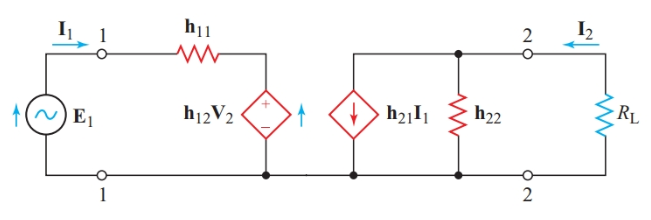# Hybrid Parameters of Two Port Network

Want create site? Find Free WordPress Themes and plugins.

For analyzing circuits containing active devices such as transistors, it is more convenient to think of the input terminals of a four-terminal coupling network as a Thévenin-equivalent voltage source and the output terminals as a Norton-equivalent current source. We then describe the coupling network in terms of four hybrid parameters (h-parameters). We determine these parameters using the same measurement techniques as for z-parameters and y-parameters.

To find the open-circuit voltage of the Thévenin-equivalent source at input terminals (port 1) in Figure 1(a), we feed V2 into the output terminals (port 2). In this circuit, we consider the Thévenin-equivalent source to be a voltage-controlled voltage source. The parameter that represents the fraction of the output voltage appearing at the input terminals is V1/V2, which is a ratio without units. This parameter is the open-circuit reverse- voltage ratio, h12.

Since we are treating the dependent source as a voltage-controlled voltage source, we short-circuit the output terminals while we measure the input voltage and current, as shown in Figure 1(b). The parameter h11 is V1/I1, which is expressed in ohms and represents the short-circuit input impedance of the network. Since h12V2 is a voltage source, the equivalent input circuit for the coupling network shows the dependent voltage source and input impedance in series, as in Figure 1(c).Figure 1 Finding the Thévenin-equivalent input circuit of a four-terminal network: (a) Open-circuit reverse voltage; (b) Internal input impedance; (c) Network input parameters

To determine the short-circuit current of the Norton-equivalent source at the output terminals (port 2) in Figure 2(a), we feed I1 into the input terminals and short-circuit the output terminals through the ammeter measuring I2. As long as the network impedances are linear (independent of voltage and current), I2 will be a constant fraction of the input current I1. The ratio I2/I1 is the short-circuit forward-current ratio, h21.Figure 2 Finding the Norton-equivalent output circuit of a four-terminal network: (a) short-circuit forward current; (b) output admittance; (c) complete hybrid parameters.

The output impedance of a Norton-equivalent source is in parallel with the current source, so the fourth hybrid parameter is expressed as an admittance. Since we are treating this dependent source as a current-controlled current source, we leave the input terminals of the network open-circuit to make I1 zero while we measure I2 and V2. The parameter h22 is I2 /V2, which is expressed in Siemens and represents the open-circuit output admittance. These equations summarize the four hybrid parameters of a four-terminal coupling network:

Short-circuit input impedance:

$\begin{matrix}{{\text{h}}_{\text{11}}}\text{=}\frac{{{\text{V}}_{\text{1}}}}{{{\text{I}}_{\text{1}}}}\left( \text{with }{{\text{V}}_{\text{2}}}\text{=0} \right) & {} & \left( 1 \right) \\\end{matrix}$

Open-circuit reverse-voltage ratio:

$\begin{matrix}{{\text{h}}_{\text{12}}}\text{=}\frac{{{\text{V}}_{\text{1}}}}{{{\text{V}}_{\text{2}}}}\left( \text{with }{{\text{I}}_{\text{1}}}\text{=0} \right)Open-Circuit & {} & \left( 2 \right) \\\end{matrix}$

Short-circuit forward-current ratio:

$\begin{matrix}{{\text{h}}_{\text{21}}}\text{=}\frac{{{\text{I}}_{\text{2}}}}{{{\text{I}}_{\text{1}}}}\left( \text{with }{{\text{V}}_{\text{2}}}\text{=0} \right)Short-Circuit & {} & \left( 3 \right) \\\end{matrix}$

$\begin{matrix}{{\text{h}}_{\text{22}}}\text{=}\frac{{{\text{I}}_{\text{2}}}}{{{\text{V}}_{\text{2}}}}\left( \text{with }{{\text{I}}_{\text{1}}}\text{=0} \right) & {} & \left( 4 \right) \\\end{matrix}$

Figure 2(c) shows the resulting h-parameter equivalent circuit. For the Thévenin-equivalent source for the network input, we can write a Kirchhoff’s voltage-law equation, as we did for z-parameters. For the Norton-equivalent source for the network output, we write a Kirchhoff’s current-law equation, as we did for y-parameters. The two unknowns in these equations are I1 and V2.

\begin{align}& \begin{matrix}{{\text{h}}_{\text{11}}}{{\text{I}}_{\text{1}}}\text{+}{{\text{h}}_{\text{12}}}{{\text{V}}_{\text{2}}}\text{=}{{\text{E}}_{\text{1}}} & {} & \left( 5 \right) \\\end{matrix} \\& \begin{matrix}{{\text{h}}_{\text{21}}}{{\text{I}}_{\text{1}}}\text{+}{{\text{h}}_{\text{22}}}{{\text{V}}_{\text{2}}}\text{=}{{\text{I}}_{\text{2}}} & {} & \left( 6 \right) \\\end{matrix} \\\end{align}

The transistor amplifier equivalent circuit of Figure 3 is a typical example of hybrid parameters.Figure 3 Hybrid parameters of a simple transistor amplifier

We cannot use Thevenin’s theorem to find the equivalent internal resistance of a dependent source when the controlling element is included in the transformation. Therefore, when we want to determine the input and output impedances of coupling networks, we must calculate V/I. We determined h11 with a short circuit across the output terminals of the network. In the circuit of Figure 3, Zin differs slightly from h11 since the circuit has some reverse coupling.

Did you find apk for android? You can find new Free Android Games and apps.